While performing deletion operation in binary search tree we come to three cases. the node that we want to delete

1. Node has no child
2. Node has exactly one child node
3. Node has exactly two child node

let's see them one by one with the help of an example

### Node has no child

For deleting a node that doesn't have any child then we replace the linked part of the node's parent node that we want to delete.

if the node is the left child node then the left child of the parent becomes None and if the node is a right child then the right child of the parent becomes None.

let's say we have a binary search tree as given below. and we want to delete node 34.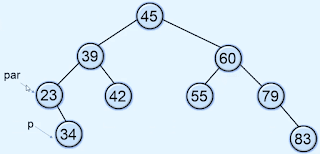to delete this node first we need to find the reference of the node that we want to delete and the reference of the parent node as you see in the image given above. and because node 34 is the right child of node 23 so we set the right child of node 23 to None.so now the 34 is deleted from the tree.

### Node has only one child

After deleting the node that has only one child then the child will come at the place of the deleted node.

let's say we have a node P that we want to delete and PR is the parent of the node and CH is the child of the node that has to be deleted.

if P is the left child of the PR then CH becomes the left child of PR and if P is the right child of PR then CH becomes the right child of PR

let's say we have a binary search tree as you see in the given image below. and we want to delete node 34 which has one child 29.to delete this node we need to find the reference of the node that we want to delete and reference its parent and child node.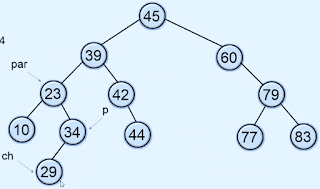because the node P that we want to delete is the right child of node 23 so we store the reference of the child node of node P into the parent node 23 as a right child.so now the 34 is deleted and the new node 29 is replaced at 34.### Node has two child

to delete a node that has two child nodes first we need to find the inorder successor of the node that we want to delete. and then copy the data of the inorder successor to the node and then delete the inorder successor from the tree.

#### How to find an Inorder successor

let's say we want to delete the node that has value 84 and to find the inorder successor of this node we need to traverse the right subtree of node 84 and pick the leftmost node in the right subtree.so the leftmost node in the right subtree of node 84 is 86 so this is the inorder successor of node 84.

let's say we have a binary search tree and we want to delete node 60 that has two child nodes as you see in the image given below.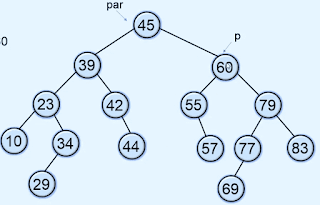so first we need to find the reference of the node, it's a parent node, and the inorder successor of the node and the parent of the inorder successor node as you see in the given below image.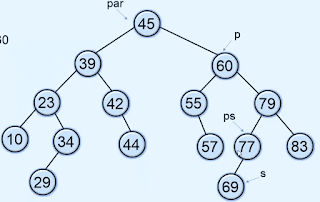and then we replace the value of the node by the inorder successor of the node as you see in the image given below we replace the value 60 by its inorder successor which is 69.and then we delete the inorder successor 69 so now the node 60 is deleted.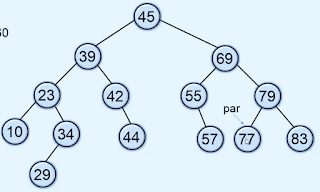#### Program to perform deletion operation in the binary search tree.

```class TreeEmptyError(Exception):
pass

class Node:
def __init__(self, value):
self.info = value
self.lchild = None        self.rchild = None

class BinarySearchTree:

def __init__(self):
self.root = None
def is_empty(self):
return self.root is None
def _insert(self, p, x):
if p is None:
p = Node(x)
elif x < p.info:
p.lchild = self._insert(p.lchild, x)
elif x > p.info:
p.rchild = self._insert(p.rchild, x)
else:
print(x, " already present in the tree")
return p

def insert1(self, x):
p = self.root
par = None        while p is not None:
par = p
if x < p.info:
p = p.lchild
elif x > p.info:
p = p.rchild
else:
print(x + " already present in the tree")
return
temp = Node(x)

if par is None:
self.root = temp
elif x < par.info:
par.lchild = temp
else:
par.rchild = temp

def search(self, x):
return self._search(self.root, x) is not None
def _search(self, p, x):
if p is None:
return None        if x < p.info:
return self._search(p.lchild, x)
if x > p.info:
return self._search(p.rchild, x)
return p

def search1(self, x):
p = self.root
while p is not None:
if x < p.info:
p = p.lchild
elif x > p.info:
p = p.rchild
else:
return True        return False
def delete(self, x):
self.root = self._delete(self.root, x)

def _delete(self, p, x):
if p is None:
return p

if x < p.info:
p.lchild = self._delete(p.lchild, x)
elif x > p.info:
p.rchild = self._delete(p.rchild, x)
else:
if p.lchild is not None and p.rchild is not None:
s = p.rchild
while s.lchild is not None:
s = s.lchild
p.info = s.info
p.rchild = self._delete(p.rchild, s.info)
else:
if p.lchild is not None:
ch = p.lchild
else:
ch = p.rchild
p = ch
return p

def delete1(self, x):
p = self.root
par = None
while p is not None:
if x == p.info:
break            par = p
if x < p.info:
p = p.lchild
else:
p = p.rchild

if p is None:
return
if p.lchild is not None and p.rchild is not None:
ps = p
s = p.rchild

while s.lchild is not None:
ps = s
s = s.lchild
p.info = s.info
p = s
par = ps

if p.lchild is not None:
ch = p.lchild
else:
ch = p.rchild

if par is None:
self.root = ch
elif p == par.lchild:
par.lchild = ch
else:
par.rchild = ch

def min1(self):
if self.is_empty():
raise TreeEmptyError("Tree is empty")
p = self.root
while p.lchild is not None:
p = p.lchild
return p.info

def max1(self):
if self.is_empty():
raise TreeEmptyError("Tee is empty")
p = self.root
while p.rchild is not None:
p = p.rchild
return p.info

def min2(self):
if self.is_empty():
raise TreeEmptyError("Tree is empty")
return self._min(self.root).info

def _min(self, p):
if p.lchild is None:
return p
return self._min(p.lchild)

def max2(self):
if self.is_empty():
raise TreeEmptyError("Tree is empty")
return self._max(self.root).info

def _max(self, p):
if p.rchild is None:
return p
return self._max(p.rchild)

def display(self):
self._display(self.root, 0)
print()

def _display(self, p, level):
if p is None:
return        self._display(p.rchild, level + 1)
print()

for i in range(level):
print("  ", end='')
print(p.info)
self._display(p.lchild, level + 1)

def preorder(self):
self._preorder(self.root)
print()

def _preorder(self, p):
if p is None:
return        print(p.info, " ")
self._preorder(p.lchild)
self._preorder(p.rchild)

def inorder(self):
self._inorder(self.root)
print()

def _inorder(self, p):
if p is None:
return        self._inorder(p.lchild)
print(p.info, " ")
self._inorder(p.rchild)

def postorder(self):
self._postorder(self.root)
print()

def _postorder(self, p):
if p is None:
return        self._postorder(p.lchild)
self._postorder(p.rchild)
print(p.info, " ")

def height(self):
return self._height(self.root)

def _height(self, p):
if p is None:
return 0
hL = self._height(p.lchild)
hR = self._height(p.rchild)

if hL > hR:
return 1 + hL
else:
return 1 + hR

###################################
bst = BinarySearchTree()

while True:
print("1.Display Tree")
print("2.Search(Iterative)")
print("3.Search(Recursive)")
print("4.Insert a new node(Iterative)")
print("5.Insert a noew node(Recursive)")
print("6.Delete a node(Iterative)")
print("7.Delete a node(Recursive)")
print("8.Find Minimum key(Iterative)")
print("9.Find Minimum key(Recursive)")
print("10.Find Maximum key(Iterative)")
print("11.Find Maximum key(Recursive)")
print("12.Preorder Traversal")
print("13.Inorder Traversal")
print("14.Postoder Traversal")
print("15.Height of tree")
print("16.Quit")
choice = int(input("Enter your choice : "))

if choice == 1:
bst.display()
elif choice == 2:
x = int(input("Enter the key to be searched : "))
if bst.search1(x):
print("Key found")
else:
elif choice == 3:
x = int(input("Enter the key to be searched : "))
if bst.search(x):
print("Key found")
else:

elif choice == 4:
x = int(input("Etner the key to be inserte : "))
bst.insert1(x)
elif choice == 5:
x = int(input("Enter the key to be inserted : "))
bst.insert1(x)
elif choice == 6:
x = int(input("Enter the element to be deleted : "))
bst.delete1(x)
elif choice == 7:
x = int(input("Enter the element to be deleted : "))
bst.delete(x)
elif choice == 8:
print("Minimum key is ", bst.min1())
elif choice == 9:
print("Minimum key is ", bst.min2())
elif choice == 10:
print("Maximum key is ", bst.max1())
elif choice == 11:
print("Maximum key is ", bst.max2())
elif choice == 12:
bst.preorder()
elif choice == 13:
bst.inorder()
elif choice == 14:
bst.postorder()
elif choice == 15:
print("Height of tree is ", bst.height())
elif choice == 16:
break    else:
print("wrong choice")
print()```